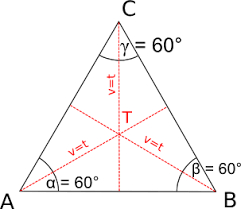# Equilateral 73374

The axial section of the cone is an equilateral triangle with a side of 12 cm. Surface and volume calculation.

V =  391.7807 cm3
S =  339.292 cm2

### Step-by-step explanation:Did you find an error or inaccuracy? Feel free to write us. Thank you!

Tips for related online calculators
Need help calculating sum, simplifying, or multiplying fractions? Try our fraction calculator.
Calculation of an equilateral triangle.